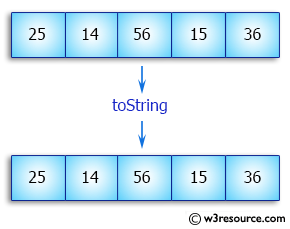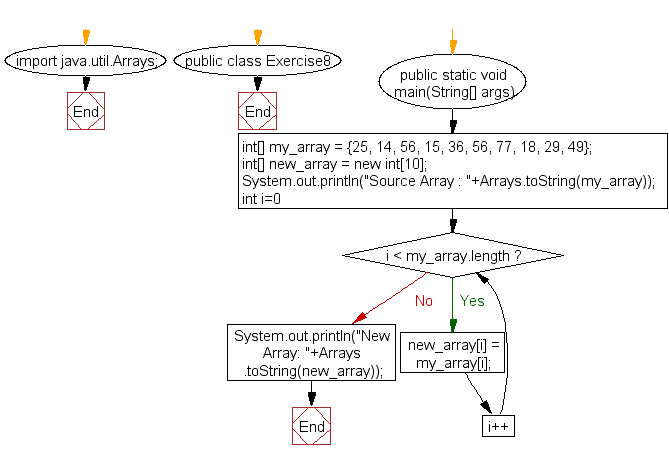﻿ Java exercises: Copy an array by iterating the array - w3resource# Java Array Exercises: Copy an array by iterating the array

## Java Array: Exercise-8 with Solution

Write a Java program to copy an array by iterating the array.

Pictorial Presentation:Sample Solution:

Java Code:

``````import java.util.Arrays;
public class Exercise8 {
public static void main(String[] args) {
int[] my_array = {25, 14, 56, 15, 36, 56, 77, 18, 29, 49};
int[] new_array = new int;

System.out.println("Source Array : "+Arrays.toString(my_array));

for(int i=0; i < my_array.length; i++) {
new_array[i] = my_array[i];
}
System.out.println("New Array: "+Arrays.toString(new_array));
}
}
```
```

Sample Output:

```Source Array : [25, 14, 56, 15, 36, 56, 77, 18, 29, 49]
New Array: [25, 14, 56, 15, 36, 56, 77, 18, 29, 49]
```

Flowchart:Visualize Java code execution (Python Tutor):

Java Code Editor:

Improve this sample solution and post your code through Disqus

What is the difficulty level of this exercise?

Test your Programming skills with w3resource's quiz.

﻿

## Java: Tips of the Day

getEnumMap

Converts to enum to Map where key is the name and value is Enum itself.

```public static <E extends Enum<E>> Map<String, E> getEnumMap(final Class<E> enumClass) {
return Arrays.stream(enumClass.getEnumConstants())
.collect(Collectors.toMap(Enum::name, Function.identity()));
}
```

Ref: https://bit.ly/3xXcFZt